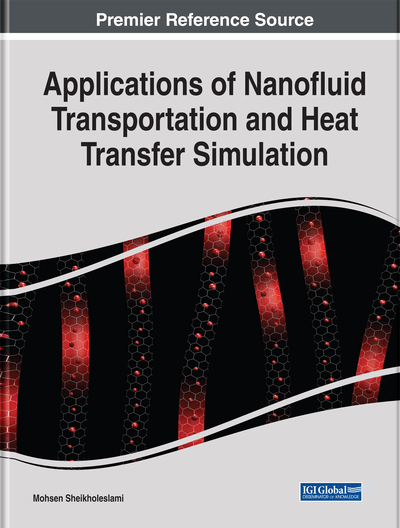# Nanotechnology as Effective Passive Technique for Heat Transfer Augmentation

DOI: 10.4018/978-1-5225-7595-5.ch001

## Abstract

Nanofluids are fluids containing the solid nanometer-sized particle dispersion. Two main methods are introduced in this chapter, namely single-phase and two-phase modeling. In first method, the combination of nanoparticle and base fluid is considered as a single-phase mixture with steady properties, and in the second method, the nanoparticle properties and behaviors are considered separately from the base fluid properties and behaviors. Moreover, nanofluid flow and heat transfer can be studied in the presence of thermal radiation, electric field, magnetic field, and porous media. In this chapter, a definition of nanofluid and its applications have been presented.
Chapter Preview
Top

## 1. Introduction

Nanofluids are produced by dispersing the nanometer-scale solid particles into base liquids with low thermal conductivity such as water, ethylene glycol (EG), oils, etc. Control of heat transfer in many energy systems is crucial due to the increase in energy prices. In recent years, nanofluids technology is proposed and studied by some researchers experimentally or numerically to control heat transfer in a process. The nanofluid can be applied to engineering problems, such as heat exchangers, cooling of electronic equipment and chemical processes. There are two ways for simulation of nanofluid: single phase and two phase. In first method, researchers assumed that nanofluids treated as the common pure fluid and conventional equations of mass, momentum and energy are used and the only effect of nanofluid is its thermal conductivity and viscosity which are obtained from the theoretical models or experimental data. These researchers assumed that nanoparticles are in thermal equilibrium and there aren’t any slip velocities between the nanoparticles and fluid molecules, thus they have a uniform mixture of nanoparticles. In second method, researchers assumed that there are slip velocities between nanoparticles and fluid molecules. So the volume fraction of nanofluids may not be uniform anymore and there would be a variable concentration of nanoparticles in a mixture. There are several numerical and semi analytical methods which have been used by several authors in order to simulate nanofluid flow and heat transfer.

### 1.1. Definition of Nanofluid

Low thermal conductivity of conventional heat transfer fluids such as water, oil, and ethylene glycol mixture is a serious limitation in improving the performance and compactness of many engineering equipment such as heat exchangers and electronic devices. To overcome this disadvantage, there is strong motivation to develop advanced heat transfer fluids with substantially higher conductivity. An innovative way of improving the thermal conductivities of fluids is to suspend small solid particles in the fluid. Various types of powders such as metallic, non-metallic and polymeric particles can be added into fluids to form slurries. The thermal conductivities of fluids with suspended particles are expected to be higher than that of common fluids. Nanofluids are a new kind of heat transfer fluid containing a small quantity of nanosized particles (usually less than 100 nm) that are uniformly and stably suspended in a liquid. The dispersion of a small amount of solid nanoparticles in conventional fluids changes their thermal conductivity remarkably. Compared to the existing techniques for enhancing heat transfer, the nanofluids show a superior potential for increasing heat transfer rates in a variety of cases (Khanafer, Vafai, & Lightstone, 2003).

### 1.2. Model Description

In the literature, convective heat transfer with nanofluids can be modeled using mainly the two-phase or single approach. In the two-phase approach, the velocity between the fluid and particles might not be zero (Li, 2000) due to several factors such as gravity, friction between the fluid and solid particles, Brownian forces, Brownian diffusion, sedimentation and dispersion. In the second approach, the nanoparticles can be easily fluidized and therefore, one may assume that the motion slip between the phases, if any would be considered negligible (Xuan & Roetzel, 2000). The latter approach is simpler and more computationally efficient.

## Complete Chapter List

Search this Book:
Reset